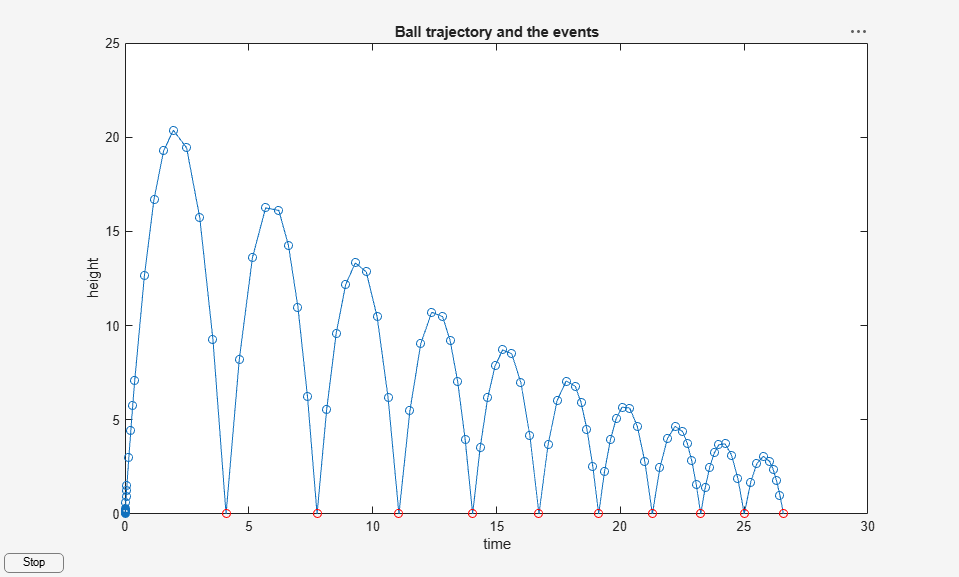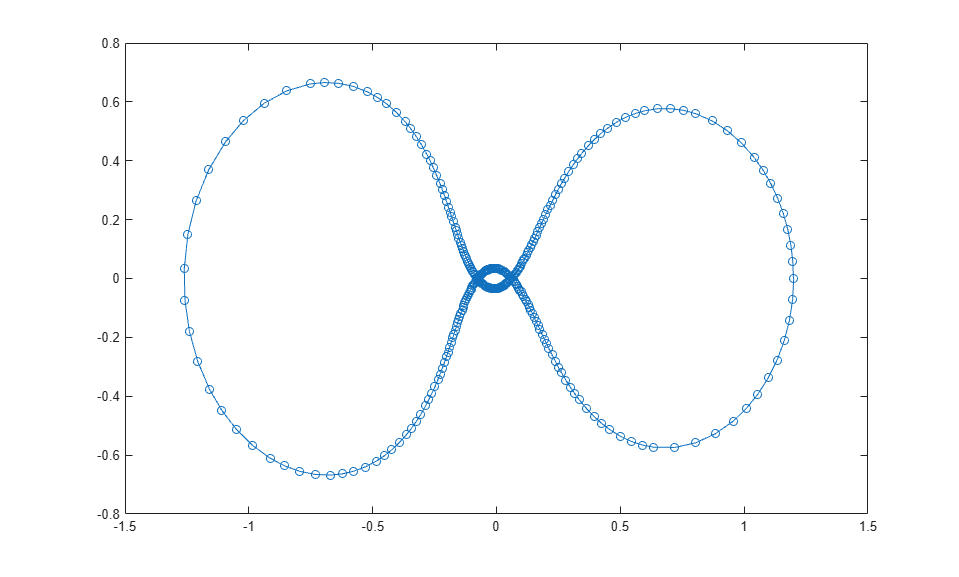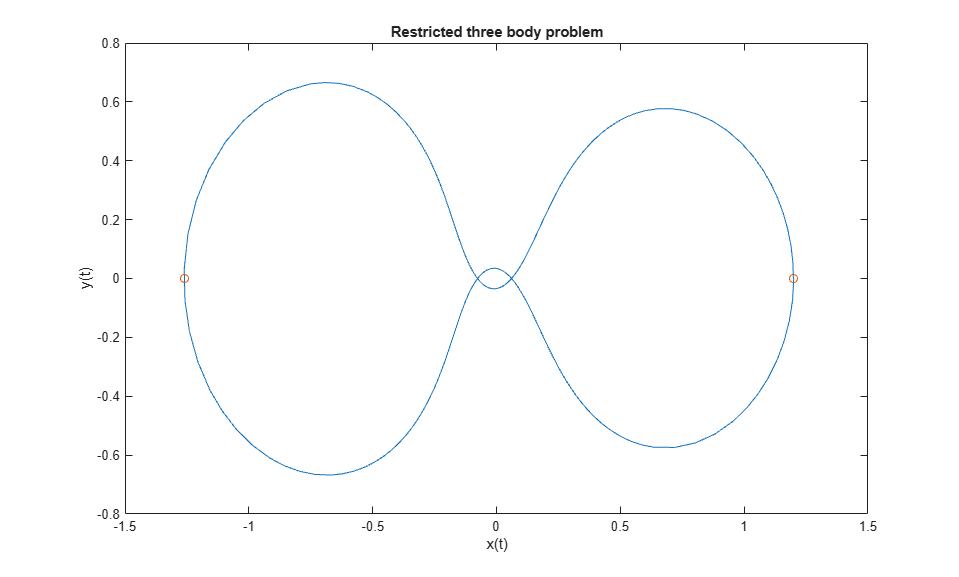## ODE Event Location

### What is Event Location?

Part of the difficulty in solving some systems of ODEs is determining an appropriate time to stop the solution. The final time in the interval of integration might be defined by a specific event and not by a number. An example is an apple falling from a tree. The ODE solver should stop once the apple hits the ground, but you might not know when that event would occur beforehand. Similarly, some problems involve events that do not terminate the solution. An example is a moon orbiting a planet. In this case you might not want to stop the integration early, but you still want to detect each time the moon completes one period around the larger body.

Use event functions to detect when certain events occur during the solution of an ODE. Event functions take an expression that you specify, and detect an event when that expression is equal to zero. They can also signal the ODE solver to halt integration when they detect an event.

### Writing an Event Function

Use the 'Events' option of the odeset function to specify an event function. The event function must have the general form

[value,isterminal,direction] = myEventsFcn(t,y)

In the case of ode15i, the event function must also accept a third input argument for yp.

The output arguments value, isterminal, and direction are vectors whose ith element corresponds to the ith event:

• value(i) is a mathematical expression describing the ith event. An event occurs when value(i) is equal to zero.

• isterminal(i) = 1 if the integration is to terminate when the ith event occurs. Otherwise, it is 0.

• direction(i) = 0 if all zeros are to be located (the default). A value of +1 locates only zeros where the event function is increasing, and -1 locates only zeros where the event function is decreasing. Specify direction = [] to use the default value of 0 for all events.

Again, consider the case of an apple falling from a tree. The ODE that represents the falling body is

$y\text{'}\text{'}=-1+y{\text{'}}^{\text{\hspace{0.17em}}2}\text{\hspace{0.17em}},$

with the initial conditions $y\left(0\right)=1$ and $y\text{'}\left(0\right)=0$. You can use an event function to determine when $y\left(t\right)=0$, which is when the apple hits the ground. For this problem, an event function that detects when the apple hits the ground is

function [position,isterminal,direction] = appleEventsFcn(t,y) position = y(1); % The value that we want to be zero isterminal = 1; % Halt integration direction = 0; % The zero can be approached from either direction

### Event Information

If you specify an events function, then call the ODE solver with three extra output arguments, as

[t,y,te,ye,ie] = odeXY(odefun,tspan,y0,options)

The three additional outputs returned by the solver correspond to the detected events:

• te is a column vector of the times at which events occurred.

• ye contains the solution value at each of the event times in te.

• ie contains indices into the vector returned by the event function. The values indicate which event the solver detected.

Alternatively, you can call the solver with a single output, as

sol = odeXY(odefun,tspan,y0,options)

In this case, the event information is stored in the structure as sol.te, sol.ye, and sol.ie.

### Limitations

The root-finding mechanism employed by the ODE solver in conjunction with the event function has these limitations:

• If a terminal event occurs during the first step of the integration, then the solver registers the event as nonterminal and continues integrating.

• If more than one terminal event occurs during the first step, then only the first event registers and the solver continues integrating.

• Zeros are determined by sign crossings between steps. Therefore, zeros of functions with an even number of crossings between steps can be missed.

If the solver steps past events, try reducing RelTol and AbsTol to improve accuracy. Alternatively, set MaxStep to place an upper bound on the step size. Adjusting tspan does not change the steps taken by the solver.

### Simple Event Location: A Bouncing Ball

This example shows how to write a simple event function for use with an ODE solver. The example file ballode models the motion of a bouncing ball. The events function halts the integration each time the ball bounces, and the integration then restarts with new initial conditions. As the ball bounces, the integration stops and restarts several times.

The equations for the bouncing ball areA ball bounce occurs when the height of the ballis equal to zero after decreasing. An events function that codes this behavior is

function [value,isterminal,direction] = bounceEvents(t,y) value = y(1); % Detect height = 0 isterminal = 1; % Stop the integration direction = -1; % Negative direction only 

Type ballode to run the example and illustrate the use of an events function to simulate the bouncing of a ball.

ballode### Advanced Event Location: Restricted Three Body Problem

This example shows how to use the directional components of an event function. The example file orbitode simulates a restricted three body problem where one body is orbiting two much larger bodies. The events function determines the points in the orbit where the orbiting body is closest and farthest away. Since the value of the events function is the same at the closest and farthest points of the orbit, the direction of zero crossing is what distinguishes them.

The equations for the restricted three body problem arewhereThe first two solution components are coordinates of the body of infinitesimal mass, so plotting one against the other gives the orbit of the body.

The events function nested in orbitode.m searches for two events. One event locates the point of maximum distance from the starting point, and the other locates the point where the spaceship returns to the starting point. The events are located accurately, even though the step sizes used by the integrator are not determined by the locations of the events. In this example, the ability to specify the direction of the zero crossing is critical. Both the point of return to the starting point and the point of maximum distance from the starting point have the same event values, and the direction of the crossing is used to distinguish them. An events function that codes this behavior is

function [value,isterminal,direction] = orbitEvents(t,y) % dDSQdt is the derivative of the equation for current distance. Local % minimum/maximum occurs when this value is zero. dDSQdt = 2 * ((y(1:2)-y0(1:2))' * y(3:4)); value = [dDSQdt; dDSQdt]; isterminal = [1; 0]; % stop at local minimum direction = [1; -1]; % [local minimum, local maximum] end 

Type orbitode to run the example.

orbitode 
This is an example of event location where the ability to specify the direction of the zero crossing is critical. Both the point of return to the initial point and the point of maximum distance have the same event function value, and the direction of the crossing is used to distinguish them. Calling ODE45 with event functions active... Note that the step sizes used by the integrator are NOT determined by the location of the events, and the events are still located accurately.